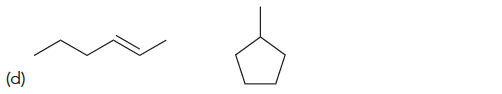Organic Chemistry Practice Problems What is the Relationship Between Isomers? Practice Problems Solution: For each of the following pairs of compounds, dete...

🤓 Based on our data, we think this question is relevant for Professor C. Gonzalez's class at FIU.

# Solution: For each of the following pairs of compounds, determine the relationship between the two compounds:

###### Problem

For each of the following pairs of compounds, determine the relationship between the two compounds:What is the Relationship Between Isomers?

What is the Relationship Between Isomers?

#### Q. For each of the following pairs of compounds, determine the relationship between the two compounds:

Solved • Fri May 27 2016 07:38:58 GMT-0400 (EDT)

What is the Relationship Between Isomers?

#### Q. For each of the following pairs of compounds, determine the relationship between the two compounds:

Solved • Fri May 27 2016 07:37:48 GMT-0400 (EDT)

What is the Relationship Between Isomers?

#### Q. For each of the following pairs of compounds, determine the relationship between the two compounds:

Solved • Fri May 27 2016 07:36:48 GMT-0400 (EDT)

What is the Relationship Between Isomers?

#### Q. For each of the following pairs of compounds, determine the relationship between the two compounds:

Solved • Fri May 27 2016 07:35:36 GMT-0400 (EDT)